# Introduction to Stack Data Structure

## Introduction to Stack Data Structure:

In this article, I will give you an introduction to the Stack Data Structure and we will discuss some of its applications. Then we will discuss the abstract data type of stack i.e. Stack ADT.

##### Stack Data Structure:

Stack is a data structure that works on LIFO (last in first out) principle. A stack is a collection of elements or sets of values. And we can insert or delete that collection element by following the LIFO principle.

Suppose, you have inserted 10 elements and then you want to delete some elements, so which element will be deleted? Only the last element or the element which was inserted at last is going to be deleted first. We use this type of mechanism in our day-to-day life at many places. Whatever we do manually, we want to automate or computerized that one. So, we write the procedures or programs for those things, so that our computer can perform those tasks. Let us see a few examples of where the stack is used in our daily life.

#### Stack Applications in Real-Time:

If suppose we have a car park area of a single row and one end of the row is closed or a dead-end where there is no exit. Cars can only enter and exit the row at one end. If we want to park our car in a specific spot in the row, but there is no available spot near it, we could use a stack to keep track of our movements.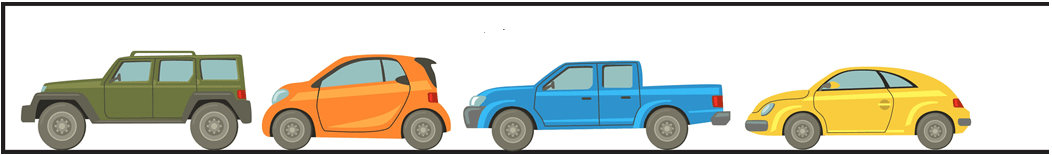Here, 4 cars are parked. These cars have entered from the right side and there is a dead end on the left side. If we want to take out these cars, which direction should we go to get out? As you can see, the left side is closed, so we will have to take out these cars in the same direction from which they entered.

So which car was inserted last? Yellow car. Which car will go out first? Yellow car. So last in will go out first. Since the first car that entered will be the last one to be taken out. The green car will be taken out at last. Let us see another example.

##### Can of Balls:

Let us say we have a can that can hold three balls, and we want to stack the balls in the can. We first put the first ball in the can at the bottom, then the second ball on top of the first one, and finally, the third ball on top of the second one as shown in the below image.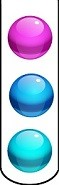From which directions do we insert these balls? As the bottom of the container is closed so we have to insert these balls from the open direction that is from the top of the container. If we want to take out the balls, we will take out these balls in the same direction from which they were inserted which is from the top of the container.

So, which is the last ball put in this stack? Pink ball. We should take out the pink ball first. The recently inserted item in the stack will become the first item of the stack to be removed. So first in what sense? Here first means the item which will come out first from the stack. So, the can of balls is a stack.

We can take the example of a stack of plates or books, a stack of paper bags, etc. We cannot take out products from the middle or from the bottom. We have to take all the products from the top.

We have already seen the recursion topic in our previous articles. Recursions are the functions that call themselves. So, their behavior is like a loop but they internally use a stack. We have already studied these things in detail. So, recursion uses the stack. Now let us see the ADT (Abstract Data Type) of the stack.

Stack Abstract data type contains data representation and the operations on the stack. ADT just gives the definition of the stack in terms of data representation and operations.

###### Data:
1. Space for storing elements.
2. Top Pointer
###### Operations:
1. push (x)
2. pop ()
3. peek (index)
4. stackTop ()
5. isEmpty ()
6. isFull ()

In data, we require space for storing the elements. As we said a stack is a collection of elements. So, we need space for storing those collections of elements. The next thing in data is, we need a top pointer that is pointing to the topmost element in the stack. Because the topmost element is the important one because we have to keep updating this pointer after every insertion or deletion in the stack.

##### Insert Operation on Stack:

In operations, we have push(x) that will insert the value x in the stack. Pop will delete the topmost element of the stack. Peek will look for the given value. Let us see all the operations with an example. Please have a look at the following stack.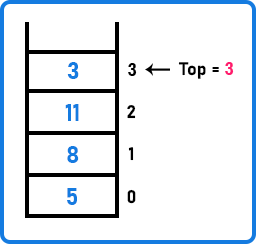Here, we have a stack, let us call it S and the top is pointing to value 3. The recently inserted value is 3. Now let us insert a new element. For inserting a new element, we will use a function that is push(x). As we know that insertion and deletion are performed at the top of the stack. So let us insert a value of 10,

###### S.push (10)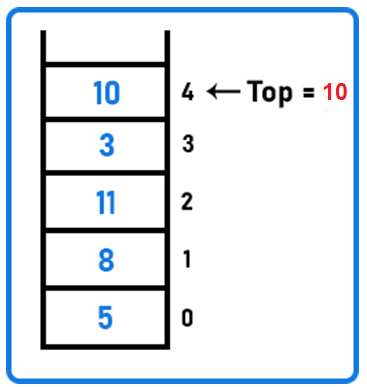Here, after inserting 10, the top pointer is updated and now pointing to value 10. Now the stack has 5 elements. So, push means to insert only one value. If you want to insert more than one value then call the push function many times as per your requirements.

##### Delete Operation on Stack:

Now let us delete or remove the topmost element from the stack. So, for this, we have a pop function.

###### S.pop();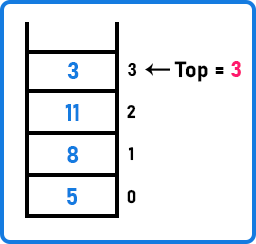After popping the topmost value, 10 is removed and the top pointer is updated and pointing to 3. The Peek function is used to know the value at a particular index. So, what is the first value here? 3 is the first value. So first is not based on the insertion, it is based on deletion. So, 11 is the 2nd value, 8 is the 3rd value and 5 is the 4th value.

Let us say we want to know the 4th value. We will get the result 5. This indicates to remove 5, we have to remove 3 values from the top of the stack as 5 is on the 3rd index. The StackTop function will return the topmost value of the stack.

S.stackTop(): It will return 3 as it is the topmost value of the stack.

isEmpty () and isFull () functions to check whether the stack is empty or full.

S.isEmpty() will return false.

S.isFull() will return false.

Now, the stack is neither empty nor full. So, both functions will return false. If we insert one more value, the stack will be full and if we delete all the values, the stack will be empty.

So, we have discussed the basic concepts of Stack ADT. We have not discussed the implementation of the stack. The Stack is a collection of elements that are stored in the LIFO principle. So, where all the elements will be stored? We have two data structures where we can store the collection of elements and that are

These are the two physical data structures. We use these two data structures for implementing the Stack Data Structure. In the next article, we will see How to Implement the Stack Data Structure using Array in C Language with Examples.